# Test: Single Correct MCQs: Mechanical Properties of Solids and Fluids | JEE Advanced

## 14 Questions MCQ Test Physics For JEE | Test: Single Correct MCQs: Mechanical Properties of Solids and Fluids | JEE Advanced

Description
Attempt Test: Single Correct MCQs: Mechanical Properties of Solids and Fluids | JEE Advanced | 14 questions in 30 minutes | Mock test for JEE preparation | Free important questions MCQ to study Physics For JEE for JEE Exam | Download free PDF with solutions
QUESTION: 1

### A vessel containing water is given a constant acceleration ‘a’  towards the right along a straight horizontal path. Which of the following diagrams in Fig. represents the surface of the liquid ?

Solution: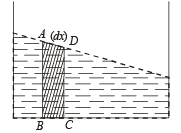Let us consider a small dotted segment of thickness dx for observation.
Since, this segment is accelerated towards right, a net force is acting in this segment towards right from the liquid towards the left of ABCD. According to Newton's third law, the segment ABCD will also apply a force on the previous section creating a pressure on it which makes the liquid rise.

QUESTION: 2

### The following four wires are made of the same material. Which of these will have the largest extension when the same tension is applied ?

Solution: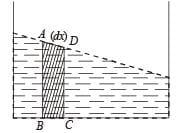QUESTION: 3

### A U-tube of  uniform cross section (see Fig) is partially filled with a liquid I. Another liquid II which does not mix with liquid I is poured into one side. It is found that the liquid levels of the two sides of the tube are the same, while the level of liquid I has risen by 2 cm. If the specific gravity of liquid I is 1.1, the specific gravity of liquid II must be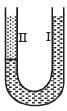Solution:

Pressure in limb I at B = Pressure in limb II at A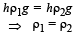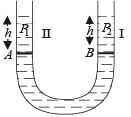QUESTION: 4

A homogeneous solid cylinder of length L (L < H/2), crosssectional area A/5 is immersed such that it floats with its axis vertical at the liquid-liquid interface with length L/4 in the denser liquid as shown in the figure. The lower density liquid is open to atmosphere having pressure P0. Then density D of solid is given by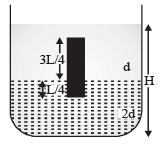Solution:

(a) Weight of cylinder = Upthrust due to upper liquid + Upthrust due to lower liquid.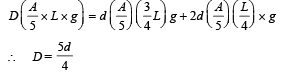QUESTION: 5

A large open tank has two holes in the wall. One is a square hole of side L at a depth y from the top and the other is a circular hole of radius R at a depth 4y from the top. When the tank is completely filled with water, the quantities of water flowing out per second from both holes are the same.
Then, R is equal to

Solution:

Equating the rate of flow, we have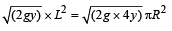[Flow = (area) × (velocity), velocity =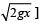where x = height from top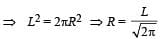QUESTION: 6

A hemispherical portion of radius R is removed from the bottom of a cylinder of radius R. The volume of the remaining cylinder is V and its mass M. It is suspended by a string in a liquid of density ρ where it stays vertical. The upper surface of the cylinder is at a depth h below the liquid surface.
The force on the bottom of the cylinder by the liquid is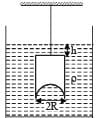Solution:

KEY CONCEPT :

According to Archimedes principle Upthrust = Wt. of fluid displaced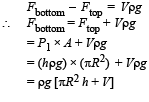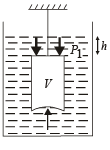QUESTION: 7

A wooden block, with a coin placed on its top, floats in water as shown in figure. The distance ℓ and h are shown here. After some time the coin  falls into the water. Then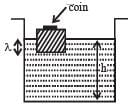Solution:

ℓ decreases as the block moves up. h will also decreases because when the coin is in water it will displace a volume of water, equal to its own volume, whereas when it is on the block it displaces more volume than to own volume (because density of coin is greater than density of water).

QUESTION: 8

The adjacent graph shows the estension (Δℓ) of a wire of length 1 m suspended from the top of a roof at one end and with a load W connected to the other end. If the crosssectional area of the wire is 10–6 m2, calculate the Young’s modulus of the material of the wire.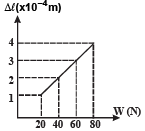Solution: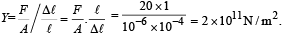QUESTION: 9

Water is filled in a contai ner upto height 3m. A small hole of area ‘a’ is punched in the wall of the container at a height 52.5 cm from the bottom. The cross sectional area of the container is A. If a/A = 0.1 then v2 is (where v is the velocity of water comin g out of th e hole)

Solution:

The square of the velocity of efflux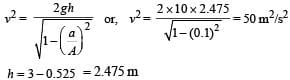QUESTION: 10

When temperature of a gas is 20ºC and pressure is changed from p1 = 1.01 × 105 Pa to p2 = 1.165 × 105 Pa then the volume changed by 10%. The bulk modulus is

Solution: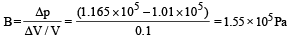QUESTION: 11

A glass tube of uniform internal radius (r) has a valve separating the two identical ends. Initially, the valve is in a tightly closed position.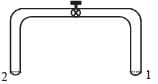End 1 has a hemispherical soap bubble of radius r. End 2 has sub-hemispherical soap bubble as shown in figure. Just after opening the valve,

Solution:

We know th at excess pressure in a soap bubble is inversely proportional to its radius. The soap bubble at end 1 has small radius as compared to the soap bubble at end 2 (given). Therefore excess pressure at 1 is more.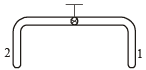As the value is opened, air flows from end 1 to end 2 and the volume of soap bubble at end 1 decreases.

QUESTION: 12

A thin uniform cylindrical shell, closed at both ends, is partially filled with water. It is floating vertically in water in half-submerged state. If ρc is the relative density of the material of the shell with respect to water, then the correct statement is that the shell is

Solution:

Let V be the volume of the material of  which the cylinder is made. The cylinder is half immersed in water.
Therefore the volume of water displaced because of the material of the cylinder is V/2. Let h be the total height of the cylinder. As the cylinder is half submerged therefore buoyant force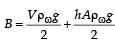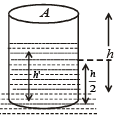where A is the area of cross-section of the cylinder
The weight of the cylinder W = Vρc g
The weight of the water inside the cylinder
= h'Aρω g
For equilibrium,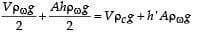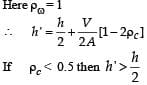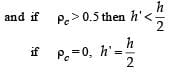QUESTION: 13

One end of a horizontal thick copper wire of length 2L and radius 2R is welded to an end of another horizontal thin copper wire of length L and radius R. When the arrangement is stretched by applying forces at two ends, the ratio of the elongation in the thin wire to that in the thick wire is

Solution: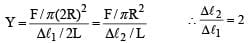QUESTION: 14

A glass capillary tube is of the shape of a truncated cone with an apex angle α so that its two ends have cross sections of different radii. When dipped in water vertically, water rises in it to a height h, where the radius of its cross section is b. If the surface tension of water is S, its density is ρ, and its contact angle with glass is θ, the value of h will be (g is the acceleration due to gravity)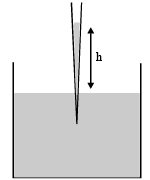Solution: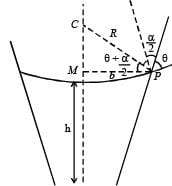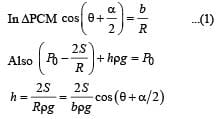Use Code STAYHOME200 and get INR 200 additional OFF Use Coupon Code